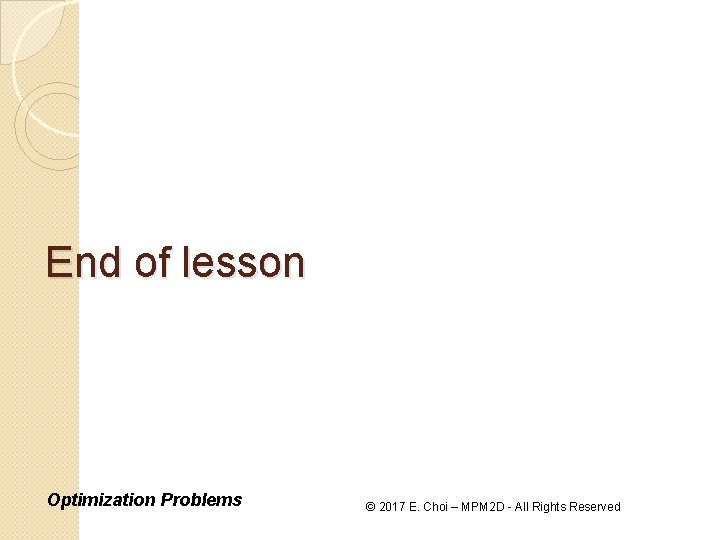• Slides: 9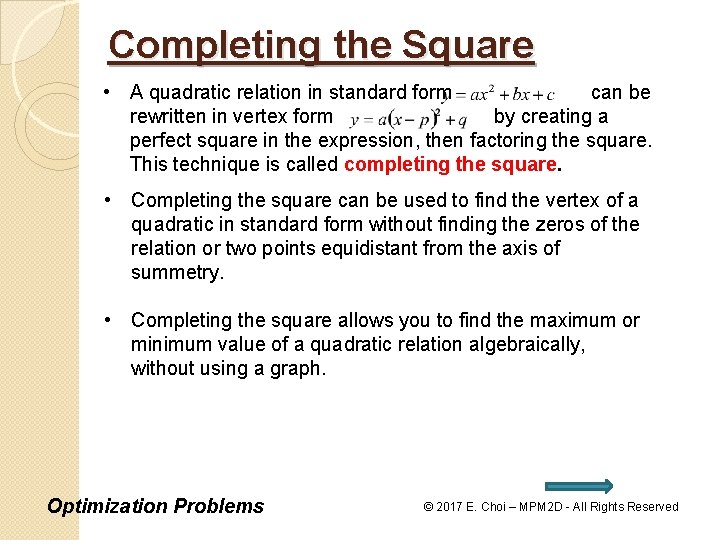Completing the Square • A quadratic relation in standard form can be rewritten in vertex form by creating a perfect square in the expression, then factoring the square. This technique is called completing the square. • Completing the square can be used to find the vertex of a quadratic in standard form without finding the zeros of the relation or two points equidistant from the axis of summetry. • Completing the square allows you to find the maximum or minimum value of a quadratic relation algebraically, without using a graph. Optimization Problems © 2017 E. Choi – MPM 2 D - All Rights ReservedCompleting the Square (Steps) 1) Remove the common constant factor from both the x and x term. 2 2) Find the constant that must be added and subtracted to create a perfect square. This value equals the square of half of the coefficient of the x –term in step 1. Rewrite the expression by adding, then subtracting, this value after the x – term inside the brackets. 3) Group the three terms that form the perfect square. Move the subtracted value outside the brackets by multiplying it by the common constant factor. 4) Factor the perfect square and collect like terms. Optimization Problems © 2017 E. Choi – MPM 2 D - All Rights Reserved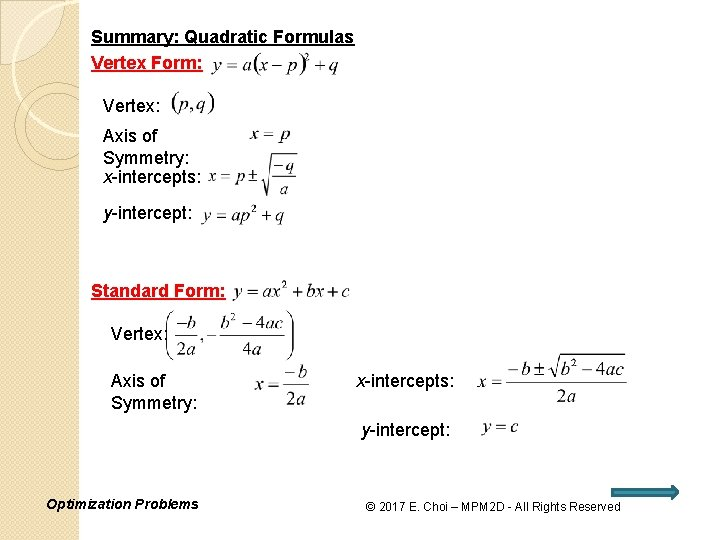Summary: Quadratic Formulas Vertex Form: Vertex: Axis of Symmetry: x-intercepts: y-intercept: Standard Form: Vertex: Axis of Symmetry: x-intercepts: y-intercept: Optimization Problems © 2017 E. Choi – MPM 2 D - All Rights ReservedExample 1: Optimization Problem (Numbers) Find the minimum product of two numbers whose difference is 8. Let x and y be the two numbers P be the product of the two numbers Minimize Product: Given: Roots: Therefore minimum product is -16 when the 2 numbers are 4 and -4. Objective Relation Factored form!! Opens up, min value occurs! Method 2: By Completing the square: Axis of symmetry: Vertex: Optimization Problems +16 -16 Vertex: Minimum value is -16 when x is 4. © 2017 E. Choi – MPM 2 D - All Rights Reserved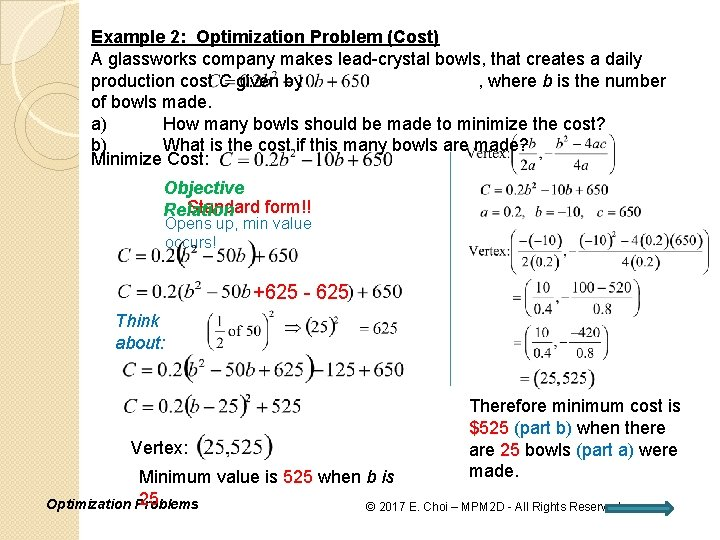Example 2: Optimization Problem (Cost) A glassworks company makes lead-crystal bowls, that creates a daily production cost C given by , where b is the number of bowls made. a) How many bowls should be made to minimize the cost? b) What is the cost if this many bowls are made? Minimize Cost: Objective Standard form!! Relation Opens up, min value occurs! +625 - 625 Think about: Vertex: Therefore minimum cost is \$525 (part b) when there are 25 bowls (part a) were made. Minimum value is 525 when b is 25. Optimization Problems © 2017 E. Choi – MPM 2 D - All Rights ReservedExample 3: Optimization Problem (Area) A rectangular fence is to be built around a playground, one side of the playground is against the school. If there is 400 m of fencing available, what dimensions would create the largest playground area? Let x and y be the dimensions of the playground in m. Maximize Area: Given: Roots: x x y Objective Relation Therefore maximum area is Factored form!! 20000 m 2 when the Opens down, dimensions are 100 m by max value occurs! 200 m. By Completing the square: Axis of symmetry: +10000 -10000 Vertex: Optimization Problems Vertex: Maximum value is 20000 when x is 100. © 2017 E. Choi – MPM 2 D - All Rights Reserved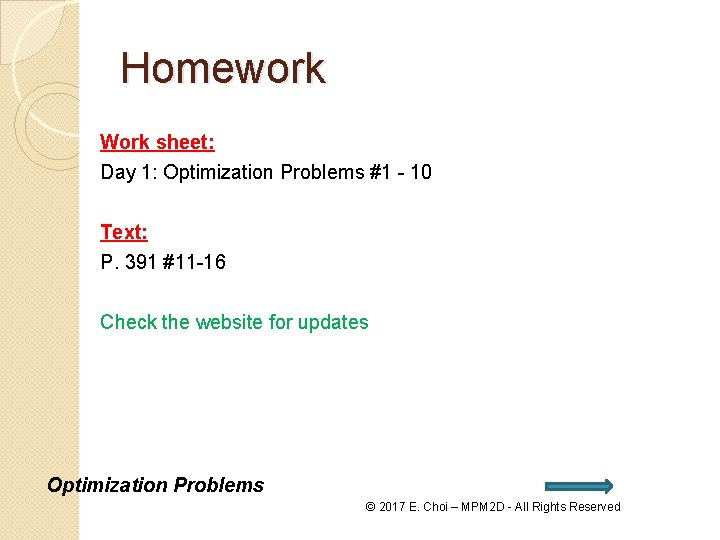Homework Work sheet: Day 1: Optimization Problems #1 - 10 Text: P. 391 #11 -16 Check the website for updates Optimization Problems © 2017 E. Choi – MPM 2 D - All Rights Reserved